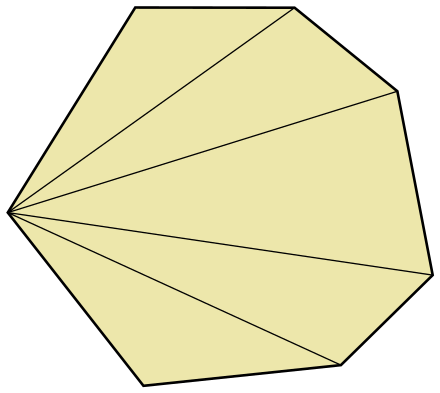# Bullet Polygonal Shapes

Is a BulletConvexHullShape the same as a CollisionPolygon?

No, neither BulletConvexHullShape nor BulletTriangleMeshShape are the same as CollisionPolygon.

Then what did it mean by adding points in the manual? Also, how to make a polygon in Bullet?

You can make a polygon out of multiple connected triangles using BulletTriangleMeshShape.

I don’t understand what you mean by your question on adding points.

https://docs.panda3d.org/1.10/python/programming/physics/bullet/collision-shapes#convex-hull-shape

``````shape2 = BulletConvexHullShape()
Point3(1, 1, 2),
Point3(0, 0, 0),
Point3(2, 0, 0),
Point3(0, 2, 0),
Point3(2, 2, 0),
])
``````

Yes, that does look like the correct way to add points to a convex hull shape.

So does this create a polygon, or no?

No, it creates a three-dimensional convex hull.

If I want to make a polygon, can I just do this?

``````p1 = Point3(0, 0, 0)
p2 = Point3(0, 0, 0)
p3 = Point3(0, 0, 0)

p4 = Point3(0, 10, 0)
p5 = Point3(0, 10, 0)
p6 = Point3(0, 10, 0)

p7 = Point3(10, 10, 0)
p8 = Point3(10, 10, 0)
p9 = Point3(10, 10, 0)

p10 = Point3(10, 0, 0)
p11 = Point3(10, 0, 0)
p12 = Point3(10, 0, 0)

triMesh = BulletTriangleMesh()

shape = BulletTriangleMeshShape(mesh, dynamic=False)
``````

Think about it: can you make a triangle out of three points that are the same? Each triangle would only be the size of a point, so you would create a shape with no area at all.

The easiest way to triangulate a convex polygon with an arbitrary number of vertices is to fan them out from a single point, like so:The following code shows how to do this (fanning out from the last point in this case):

``````points = [
Point3(0, 0, 0),
Point3(0, 10, 0),
Point3(10, 10, 0),
Point3(10, 0, 0),
]

triMesh = BulletTriangleMesh()
for pi in range(len(points) - 2):
triMesh.addTriangle(points[-1], points[pi], points[pi + 1])
``````

You might need to change the winding order if it’s ending up upside down.

If you need better topology or are dealing with non-convex polygons, you can add a center vertex and fan out from there, or even better, use Triangulator.

I don’t want to make a triangle. You see, I want a polygon in Bullet. So I thought that if I just made all the points same, it would be a super minute triangle AKA a point. And when I connect the point, it would form a polygon.

Generally speaking, shapes in modern games are composed of triangles or quads, I believe. Thus, to make up your polygon, you would likely build it out of multiple triangles.

So what if I do:

``````triMesh.addTriangle(Point3(0, 1, 0), Point3(1, 0, 0), Point3(-1, 0, 0))
# I hope you can guess the rest.
``````

I gave you the code to subdivide a polygon into triangles that together make up a single polygon. That is all you need. Is the code not working for you?

I didn’t try yet. Will try.

If you want to make a polygon, you have to “fill” the shape of the polygon with triangles → triangle mesh. If you make the triangles the size of a point you will have mesh with just infinitely small triangles apart in 4 places.

1 Like